Processing ......FreeComputerBooks.com Links to Free Computer, Mathematics, Technical Books all over the World

Concrete Mathematics: A Foundation for Computer Science (2nd Edition)
Top Free Programming Books 🌠 - 100% Free or Open Source!
• Title Concrete Mathematics: A Foundation for Computer Science (2nd Edition)
• Author(s) Ronald L. Graham, Donald Ervin Knuth, Oren Patashnik
• Publisher: Addison-Wesley Professional; 2 edition (March 10, 1994)
• Hardcover 672 pages
• eBook PDF (670 pages, 2.8 MB)
• Language: English
• ISBN-10/ASIN: 0201558025
• ISBN-13: 978-0201558029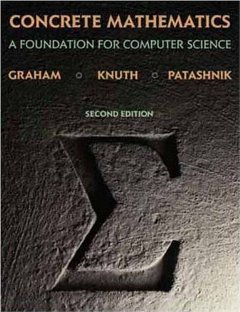Book Description

This book introduces the mathematics that supports advanced computer programming and the analysis of algorithms. The primary aim of its well-known authors is to provide a solid and relevant base of mathematical skills - the skills needed to solve complex problems, to evaluate horrendous sums, and to discover subtle patterns in data.

It is an indispensable text and reference not only for computer scientists - the authors themselves rely heavily on it! - but for serious users of mathematics in virtually every discipline.

This second edition includes important new material about mechanical summation.

• Ronald L. Graham was an American mathematician credited by the American Mathematical Society as "one of the principal architects of the rapid development worldwide of discrete mathematics in recent years".
• Donald Ervin Knuth is an American computer scientist, mathematician, and professor emeritus at Stanford University. He is the 1974 recipient of the ACM Turing Award, informally considered the Nobel Prize of computer science. Knuth has been called the "father of the analysis of algorithms".
Reviews, Ratings, and Recommendations: Related Book Categories: Read and Download Links:Similar Books:
•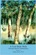A Cool Brisk Walk Through Discrete Mathematics (Stephen Davies)

This is a completely and forever free and open source educational materials dedicated to the mathematics that budding computer science practitioners actually need to know. They feature the fun and addictive teaching of award-winning lecturer!

•Mathematics for Computer Science (Eric Lehman, et al)

This book covers elementary discrete mathematics for computer science and engineering. It emphasizes mathematical definitions and proofs as well as applicable methods. explores the topics of basic combinatorics, number and graph theory, logic and proof techniques.

•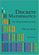Discrete Mathematics: An Open Introduction (Oscar Levin)

This is a gentle introduction to discrete mathematics. Four main topics are covered: counting, sequences, logic, and graph theory. Along the way proofs are introduced, including proofs by contradiction, proofs by induction, and combinatorial proofs.

•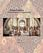Isomorphism - Mathematics of Programming (Xinyu Liu)

This book introduces the mathematics behind computer programming. It intents to tell: programming is isomorphic to mathematics. Just like in art and music, there are interesting stories and mathematicians behind the great minds.

•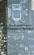Applied Discrete Structures, Fundamentals (Al Doerr, et al)

This book contains most of the fundamental concepts taught in a one semester course in discrete mathematics which is a required course for students in Computer Science, Mathematics and Information Technology.

•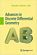Advances in Discrete Differential Geometry (Alexander I. Bobenko)

It surveys the fascinating connections between discrete models in differential geometry and complex analysis, integrable systems and applications in computer graphics and is illustrated to convince readers it's both beautiful and useful.

•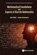Mathematical Foundations and Aspects of Discrete Mathematics

This is a book about discrete mathematics which also discusses mathematical reasoning and logic. It offers an introduction to discrete mathematics for undergraduate students in computer science and mathematics.

•A Spiral Workbook for Discrete Mathematics (Harris Kwong)

This is a book on discrete mathematics with an emphasis on motivation. It explains and clarifies the unwritten conventions in mathematics, and guides the students through a detailed discussion on how a proof is revised from its draft to a final polished form.

•Discrete Structures for Computer Science: Counting, Recursion

This book provides a broad introduction to some of the most fascinating and beautiful areas of discrete mathematical structures with examples in logic, applications of the principle of inclusion and exclusion and finally the pigeonhole principal.

•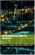Mathematics for Computer Scientists (Gareth J. Janacek, et al)

The aim of this book is to present some the basic mathematics that is needed by computer scientists. The reader is not expected to be a mathematician and we hope will find what follows useful.

•Lists, Decisions and Graphs - With an Introduction to Probability

In this book, four basic areas of discrete mathematics are presented: Counting and Listing (Unit CL), Functions (Unit Fn), Decision Trees and Recursion (Unit DT), and Basic Concepts in Graph Theory (Unit GT).

•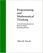Programming and Mathematical Thinking: Discrete Math & Python

Starting at an elementary level, this book teaches about fundamental structures of discrete mathematics and many simple but powerful programming techniques using those structures.

•Discrete Mathematics for Computer Science (Jean Gallier)

This book gives an introduction to discrete mathematics for beginning undergraduates. One of original features of this book is that it begins with a presentation of the rules of logic as used in mathematics. Many examples of formal and informal proofs are given.

Book Categories
 :All CategoriesTop Free BooksRecent BooksMiscellaneous BooksComputer EngineeringComputer LanguagesComputer ScienceData Science/DatabasesJava and Java EE (J2EE)Linux and UnixMathematicsMicrosoft and .NETMobile ComputingNetworking and CommunicationsSoftware EngineeringSpecial TopicsWeb Programming
Other Categories